## VECTORS AND MATRICES

This handout covers the basic vector and matrix operations that you will need for 12-271.

This handout is adapted from Chapter 9 of Pascal with Applications in Science and Engineering, Susan Finger and Ellen Finger, D. C. Heath, Lexington MA, 1986.

### 1.Vector and Matrix Operations

Every branch of engineering uses vectors and matrices. This section will discuss vector and matrix notation and illustrate some of the basic operations used with vectors and matrices.

#### 1.1 Vectors

A vector is a physical quantity that has magnitude and direction. You can visualize a vector as an arrow with a specified length pointing in a particular direction. Some examples of quantities you can represent using vectors are: the velocity of a particle specified by its speed and direction of motion, the force on a wheel specified by the magnitude and direction of the force, and the position of a planet in space specified by its distance and direction from the sun. The last vector is illustrated below: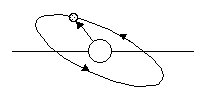Vectors are drawn as arrows because an arrow conveys the sense of direction. The tail of the vector refers to the end of the vector where the feathers of the arrow would be. The head of the vector refers to the end of the vector where the point of the arrow would be.

The magnitude of a vector is the absolute value of its length. The direction is given by the angle, in degrees or radians, from the positive x axis to the vector. A vector in polar coordinates is specified by its magnitude and angle. Polar coordinates are illustrated below: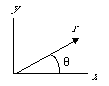A vector in polar coordinates is represented by an ordered pair of numbers. The first number is the magnitude and the second number is the angle: [r,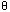]. The representation of the vector above is: [5, 36.8]; that is, you can construct the vector by going 36.8 degrees from the x axis and then going out a distance of 5 units.

You can also represent a vector in cartesian coordinates. Cartesian coordinates are named for Ren Descartes (1596-1650) a French philosopher and mathematician and a contemporary of Pascal. In cartesian coordinates a vector is represented by its projection onto the x axis and its projection onto the y axis: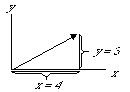Cartesian coordinates are also referred to as rectangular coordinates. The vector illustrated above is a two-dimensional vector. You can represent it by the ordered pair of numbers: [x, y]. By convention, the x coordinate is given first and the y coordinate is given second. The vector above is [4, 3]. That is, the vector goes 4 units in the x direction and 3 units in the y direction.

Not all vectors have positive coordinates. The figure below illustrates the following vectors in cartesian coordinates: [4,3], [-4,3], [-4,-3] and [4,-3]: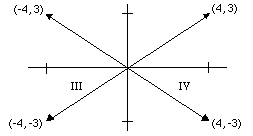You can see that the x-axis and the y-axis divide the x-y plane into 4 regions. These regions are called quadrants. The figure gives the standard names for the quadrants. The first vector [4, 3] is in quadrant I, [-4, 3] is in quadrant II, [-4,-3] is in quadrant III, and [4,-3] is in quadrant IV.

#### 1.2 Vector Notation

Because vectors are used so frequently in mathematics, science, and engineering, a compact standard notation for representing vectors has been developed. You must always specify the basis of a vector, that is the coordinate system in which the components are given. The most common basis is the cartesian coordinate system. The basis for this system in two dimensions is a vector with a length of one unit parallel to the x-axis and a vector with a length one unit parallel to the y-axis: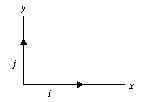You can write any two-dimensional vector in terms of the unit basis. This statement is equivalent to saying that you can represent any point in the x,y plane by its x coordinate, which is a multiple of the unit vector i, and its y coordinate, which is a multiple of the unit vector j. By convention, the vector parallel to the x-axis is called vector i, and the vector parallel to the y-axis is called vector j. For example, you can represent a two-dimensional vector, D, by its components:

D = [ d1, d2 ]

where d1 is the component along the x-axis and d2 is the component along the y-axis. You can visualize the vector, D, as:

 D D

The first cell of the array D stores the x coordinate of the vector, and the second cell stores the y coordinate.

Similarly, you can visualize a vector represented in polar coordinates as:

 D D

except the first cell now represents r, the magnitude of the vector, and the second cell represents, the angle that the vector makes with the x-axis. This example emphasizes the importance of specifying the basis of a vector. The vector (1,1) in rectangular coordinates is not the same vector as (1,1) in polar coordinates.

#### 1.3 Converting Between Coordinate Systems

Because you can perform some vector operations more easily in polar coordinates and others more easily in cartesian coordinates, you should know how to convert between coordinate systems. You can find the polar coordinates, r and, of a vector from its cartesian coordinates, x and y, by drawing a simple diagram. Referring to the figure below, you can see that the vector forms a right triangle with the x and y coordinates:

You can use the Pythagorean theorem to write the formula for the hypotenuse, r:

r  =  (x2+ y2

You can findusing the relationship:

tan= y/x

or= tan-1(y/x)

The function , tan-1, or arctan, returns the principal angle whose tangent is. The principal angle is the angle in the first or fourth quadrant whose tangent is. The vectors [4,3] and [-4,-3] will have the same arctan because y/x is equal to 4/3 in both cases. To get the correct angle for vectors in the second or third quadrant, you must addto the angle. This is equivalent to adding 180o to an angle expressed in degrees.

To convert from rectangular to polar coordinates, you must compute not only which quadrant a point is in, you must also consider what will happen if the point lies on the y axis. If the point lies on the y axis, then x is zero and the angle is "pi"/2 radians. What happens if x is equal to zero? To prevent division by zero, you must test the value of x before computing the angle. If x is zero, you must set the angle to "pi"/2.

To convert a vector in polar coordinates to cartesian coordinates, refer to the figure above again. Using the trigonometric identities, you can write x and y directly in terms of r and "theta":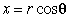and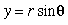#### 1.4 Multi-Dimensional Vectors

Vectors are not restricted to two dimensions. There are three-dimensional vectors, n-dimensional vectors, and infinite-dimensional vectors. While you cannot visualize vectors of more than three dimensions spatially, multi-dimensional vectors are used in many mathematical formulations of physical systems. In standard notation for multi-dimensional vectors, the components of an n-dimensional vector D, are represented by d1,d2,...dn: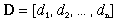Again, you must specify the basis of the vector. The standard basis is the rectangular coordinate system in which the basis vectors are mutually perpendicular vectors of unit length. There are alternate bases, such as spherical and cylindrical, but these bases are harder to generalize in higher dimensions and are difficult to work with even in three dimensions.

You can construct three-dimensional vectors that have three elements: one for the x-axis, one for the y-axis, and one for z-axis. In cartesian coordinates, three-dimensional vectors are given by their x, y, and z coordinates: [x,y,z].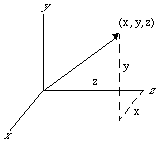#### 1.5 Scalar Multiplication of Vectors

In scalar multiplication each element of the vector is multiplied by a constant. The result of scalar multiplication is another vector that has the same direction as the original vector, but whose magnitude has been changed by the value of the constant multiplier. The figure below shows a vector that scaled first by a factor of 2, and then by a factor of -2. The vectors are offset to show them more clearly: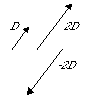If a vector D is scaled in the x and y directions by a constant, c, then the components of the new vector, D' (read as D prime), are: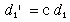and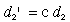You can write these equations more compactly as: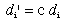for i=1,2

This equation is equivalent to stating: take every component of the vector D and multiply it by the constant c. You can write this equation even more compactly as: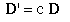When you see vector notation such as that above, you must realize that it does not represent a single multiplication. The notation means that every component of the vector D must be multiplied by the constant c to create the scaled vector D'.

#### 1.6 Vector Addition

Graphically, you can add two vectors by drawing one vector and then drawing the second vector starting from the head of the first. Draw the resultant vector between the tail of the first vector and the head of the second vector: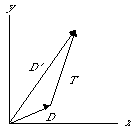Mathematically, you can perform vector by adding the corresponding components of each vector together; that is, add the x components of the two vectors together, and their sum becomes the x component of the new vector. If the two vectors are: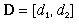and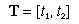then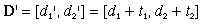You can write the addition for each component of the vector: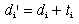for i = 1,2

Or you can write the addition in terms of the vectors themselves:Again, remember that equation above does not represent a single addition operation, but indicates that each element of the vector T should be added to the corresponding element of vector D. Notice that vector addition can only be performed if the vectors have the same number of elements.

#### 1.7 The Dot Product of Two Vectors

The dot and cross products are functions that operate on vectors in a multiplicative fashion. The definitions of these functions are, in some sense, arbitrary formulas for combining two vectors; however, both the dot product and the cross product are widely used in physics and engineering because they arise naturally in the solution of many problems involving vectors.

The dot product of two vectors, D and F, is defined to be the real number: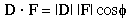where

|D| is the magnitude of the vector D
|F| is the magnitude of the vector Fis the angle between the vectors D and F.

The expression D"point"F is read as: D dot F. The dot product is a scalar function of the two vectors, because the magnitudes of the vectors are scalars as is cos(). So, the dot product of two vectors is a single real number. For this reason, the dot product is also referred to as the scalar product.

In physics, you use the dot product to compute the word. Work is defined as a force applied over a distance. To pull a massive block across a floor, you can attach a rope to the block and pull on the rope: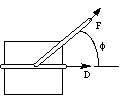The work done to move the block is the product of the force times the distance, but only the force applied in the direction of motion contributes to the work. The force shown above applied in the upward direction does not contribute to the work done in moving the block forward. As will be shown below, you can express the work as the dot product of force and distance.

To compute the amount of force that contributes to the motion, you can take the projection of the force vector onto the distance vector. You can find the projection of vector F onto the vector by dropping a line, from the head of F perpendicular to D, creating a right triangle. One side of the new triangle lies along D. The new vector is the projection of F onto D. This vector represents the force that is useful in moving the block in the direction D. From the figure, you can see that the length of the projection is |F|cos("phi").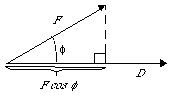If the work is defined to be the dot product of the force times the distance: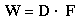or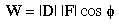then only the force that acts in the direction of motion is included in the work. The work done in moving the block is the product of the distance that the block moves and the force that acts in the direction that it moves.

An alternate definition for the dot product of two vectors uses the rectangular coordinates, x and y, of the vectors. For example, you can represent the three-dimensional vector D by its components in the x, y, and z directions: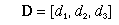In rectangular coordinates, the dot product is defined to be the sum of the products of the corresponding elements of two vectors. For a three-dimensional vector, the dot product is: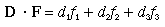or, in general, for an n-dimensional vector, the dot product is defined as: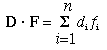From this equation, it is easy to show that the dot product is symmetric or commutative. That is: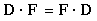You can think of the work either as the force applied in the direction of motion times the distance moved or as the distance moved in the direction of the force times the force applied. The second interpretation involves taking the projection of the distance vector onto the force vector: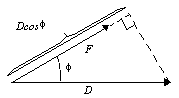Multiplying the magnitude of the projection by the magnitude of the force results in the same value for the work as the value obtained before.

From the equation above, you can show that the dot product of two perpendicular vectors is zero. This result follows directly from the fact that the cos(90) is equal to zero. Applying this result to the problem of determining the work done by a force, suppose you push down (apply a downward force) on the block. No matter how hard you push, the block will not move forward. The downward force is perpendicular to the direction of motion, so no work is performed.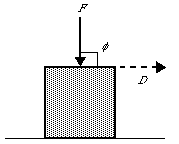Another property of the dot product is that the dot product of a vector with itself is the square of the magnitude of the vector. This property can be directly from either formula above. Using

the first formula, D"dot"D is: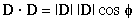Since a vector makes an angle of 0 degree with itself, this becomes: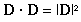You can use this equation as an alternate definition of the magnitude of a vector: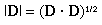#### 1.8 The Cross Product

The cross product or vector product of two vectors, R and F, is a vector that is mutually perpendicular to the two vectors, with a magnitude of |R||F|sin("phi"), where "phi" is the angle between R and F.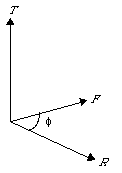The cross product operation is represented by an x, and the expression R x F is read as: R cross F. You can find the direction of the vector product, T, using the right-hand rule. For R x F, curl your fingers from the vector R to the vector F and your thumb will point in the direction of their cross product: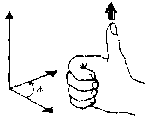Learning the right-hand rule has practical applications since most screws and threads are right-handed. If you want to unscrew a light bulb, point your right thumb in the direction that you want the bulb to go. Curl your fingers, as illustrated above. Turn the bulb in the direction that your fingers curl, and the bulb will move in the direction that your thumb points.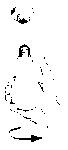The magnitude and direction of the cross product vector given above are sufficient to specify the vector; however, you can also define the cross product the vector elements. If T is the cross product of R and F, that is, if T = R x F, the elements of the vector T are defined to be: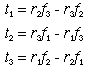From the definition, you can see that the cross product is valid only for three-dimensional vectors.

The cross product is not symmetric. Reversing the order of the vectors changes the sign of the resulting vector. The direction of the cross product, F x R, can be found by curling your fingers from F to R as illustrated below: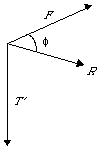The resulting vector points in the opposite direction from the vector R x F. Mathematically, R x F = -(R x F).

A common phenomenon that can be described using the cross product is torque. When you use a wrench to remove a bolt, you fix one end of the wrench to the bolt, and apply a force to the other end of the wrench. This force creates a torque: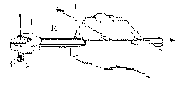By definition, the torque, "tau", of a force about a point is the vector R x F where R is the distance vector from the point to the force: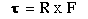### 2. Matrices

You can use a matrix to represent several vectors in a single mathematical structure. A matrix is a multi-dimensional array of numbers. For example, the two-dimensional matrix below is an array of 3 by 4 real numbers: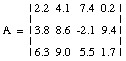By convention, a matrix that has m rows and n columns is called an m by n matrix. So the matrix above is a 3 by 4 matrix. You can think of the matrix above as a stack of 3 four-dimensional vectors.

The elements of a matrix are specified by their row and column number: "a sub i,j". As with arrays, the row number is designated as i and the column number as j. For example, in the matrix above the element "a sub 3,2" has a value of 9.0. You can find this value by going down 3 rows and over 2 columns. The general form for an m by n matrix is: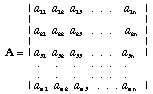Notice that a vector is just a special case of a matrix. That is, a row vector is a 1 by n matrix and a column vector is an m by 1 matrix.

#### 2.1 Scalar Multiplication of Matrices

In scalar multiplication of a matrix, each element is multiplied by a single constant value. If the matrix is R, and the scale factor is c, the elements in the new matrix R' are: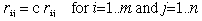Or, in matrix notation: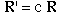The notation above indicates that you perform n by m multiplications, one for each element in the matrix. Notice that this equation looks exactly the same as the equation for scalar vector multiplication. Since a vector is a one-dimensional matrix, it is reasonable to express scalar multiplication the same way for both vectors and matrices.

#### 2.2 Matrix Addition

In matrix addition the corresponding elements of matrices are added together. Matrix addition is defined only for matrices that have the same dimensions. You cannot add a 2 by 2 matrix to a 10 by 10 matrix. If you add the elements of the matrix X to the matrix R, the the elements of the new matrix, R', are: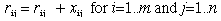Or in matrix notation: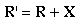#### 2.3 Matrix Multiplication

Matrix multiplication is a common operation. If you have never seen matrix multiplication before, it may take a while before it makes sense, but because you will use it so frequently in your work, it is worth the time to learn. In matrix notation, multiplication is written as: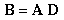However, matrix multiplication is defined only for particular pairs of matrices: those in which the number of columns in the first matrix, A, is equal to the number of rows in the second matrix, D.

In matrix multiplication, you multiply the elements of one row of a matrix by the elements of one column of the other matrix. Sum the products and the result becomes the row,column element of the new matrix. If A is a m x n matrix and D is a n x l matrix, the elements of the new matrix are given by: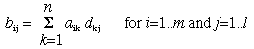To find the product of the two matrices below: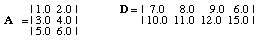start by finding the value for "b sub 11". Rewrite the summation above substituting 1 for i and 1 for j. The limit of the sum, n, has a value of 2 because there are two columns in matrix A and 2 rows in matrix D: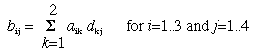When k is equal to 1, the element "a sub 11" which has a value of 1.0 is multiplied by the element "d sub 11" which has a value of 7. So, 7 becomes the current value of the running total. When k is 2, the element "a sub 12" which has a value of 2.0 is multiplied by "d sub 21" which has a value of 10.0. Add the resulting value of 20 to the running total. When k is equal to 2, you have calculated all the terms in the summation, so assign the resulting value, 27, to "b sub 11". "b sub 12" is equal to "a sub 11""d sub 12" + "a sub 12""d sub 22", or (1.0)(8.0) + (2.0)(11.0) = 30. "b sub 13" is equal to "a sub 11""d sub 13" + "a sub 12""d sub 23", or (1.0)(9.0) + (2.0)(12.0) = 33. If you locate each number in the matrices as you multiply and add, you will notice a pattern going across the rows of the first matrix and down the columns of the second matrix. To be sure that you understand the algorithm, you can verify the remainder of the elements in the B matrix.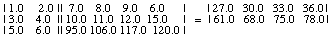Note that a 3 by 2 matrix multiplied by a 2 by 4 matrix results in a 3 by 4 matrix.

Under addition, matrices are associative and commutative. The additive inverse is -A, and 0 is a matrix with 0 for every element:

Associative: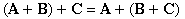Commutative: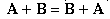Additive Inverse: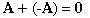Under multiplication, matrices are associative, but they are not commutative.

Not Commutative: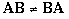Multiplicative Inverse: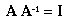The multiplicative inverse is A"sup -1", and I is a square matrix with ones on the diagonal and zeros everywhere else.

sfinger@ri.cmu.edu